# Chapter 5 Matter in Motion Measuring Motion Motion

• Slides: 40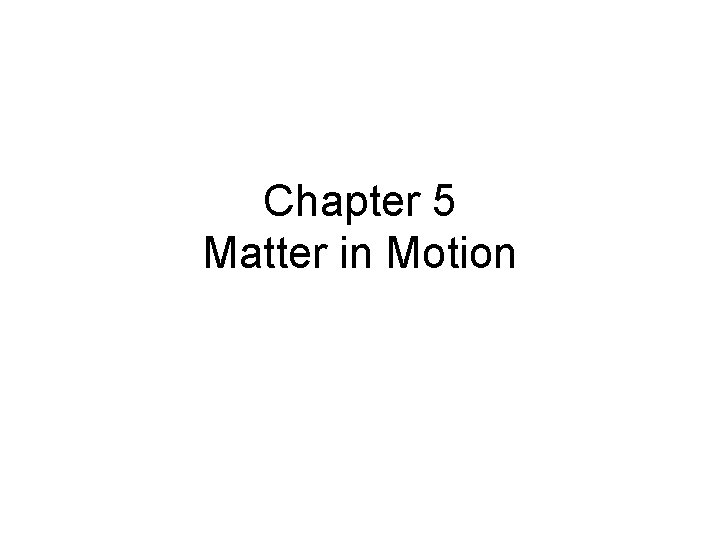Chapter 5 Matter in MotionMeasuring Motion • Motion is all around us (the moon is circling the earth, blood is traveling through your veins, tiny air particles are moving through the air) • Observing motion – To observe motion you must observe the object in relation to another object that appears to stay in place. – When an object changes position over time when compared with a reference point the object is in motion.Common Reference Points • The Earth’s surface is a common reference point for determining position and motion. • Non-moving objects such as buildings, trees, and mountains are also useful reference points.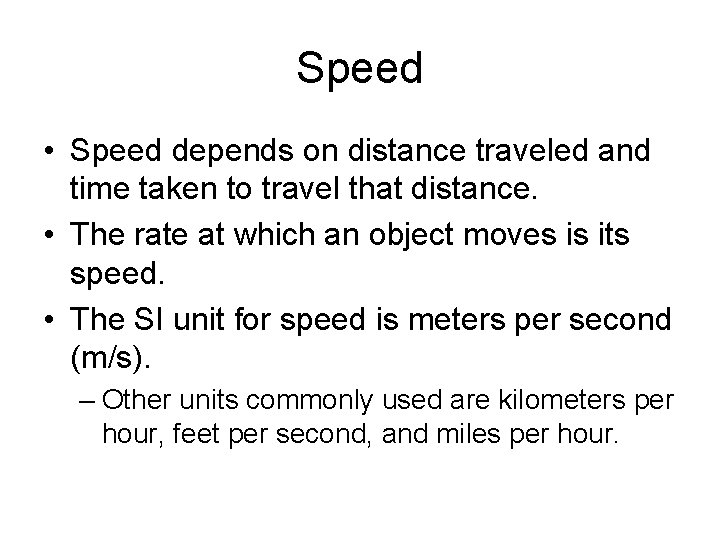Speed • Speed depends on distance traveled and time taken to travel that distance. • The rate at which an object moves is its speed. • The SI unit for speed is meters per second (m/s). – Other units commonly used are kilometers per hour, feet per second, and miles per hour.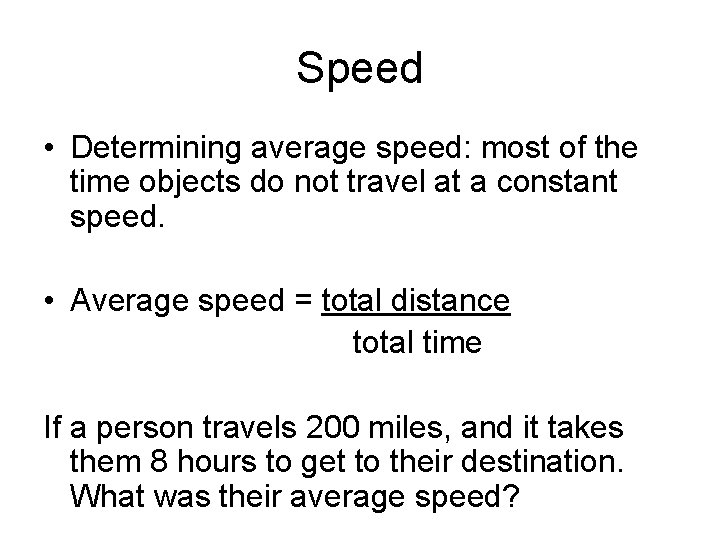Speed • Determining average speed: most of the time objects do not travel at a constant speed. • Average speed = total distance total time If a person travels 200 miles, and it takes them 8 hours to get to their destination. What was their average speed?Velocity • The speed of an object in a particular direction. • Velocity must include direction: – ie) It would not be correct to say the planes velocity is 600 km/h (that is its speed). It’s velocity would be 600 km/h south. – Velocity always includes a reference to direction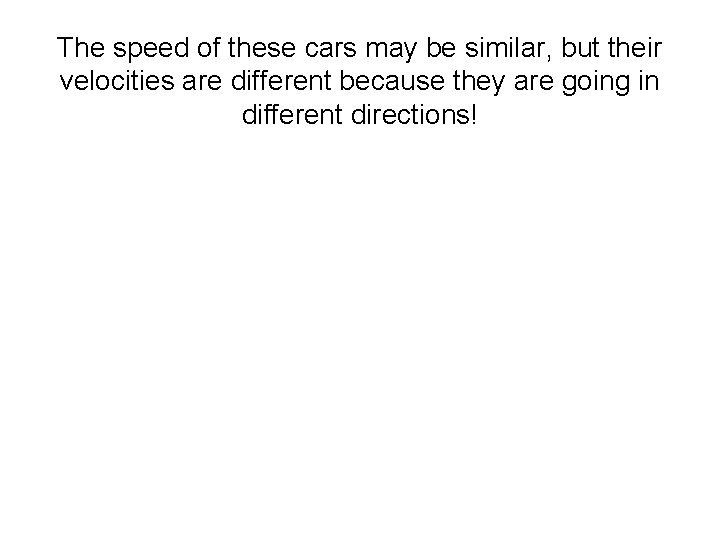The speed of these cars may be similar, but their velocities are different because they are going in different directions!Velocity changes as speed or direction changes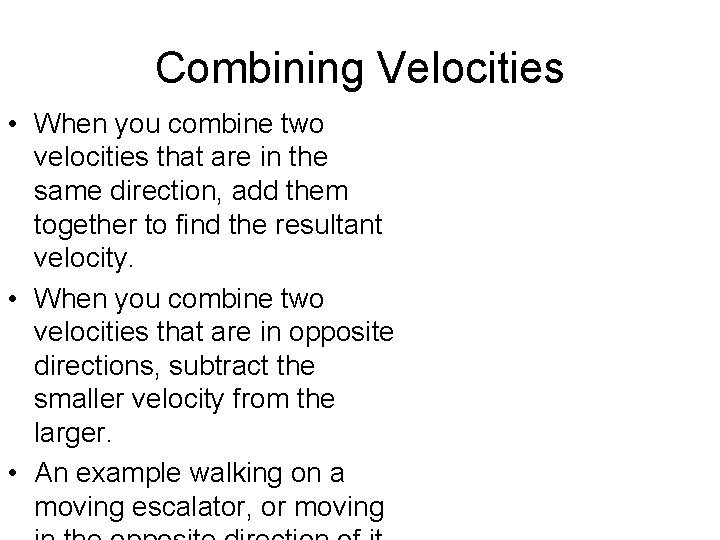Combining Velocities • When you combine two velocities that are in the same direction, add them together to find the resultant velocity. • When you combine two velocities that are in opposite directions, subtract the smaller velocity from the larger. • An example walking on a moving escalator, or moving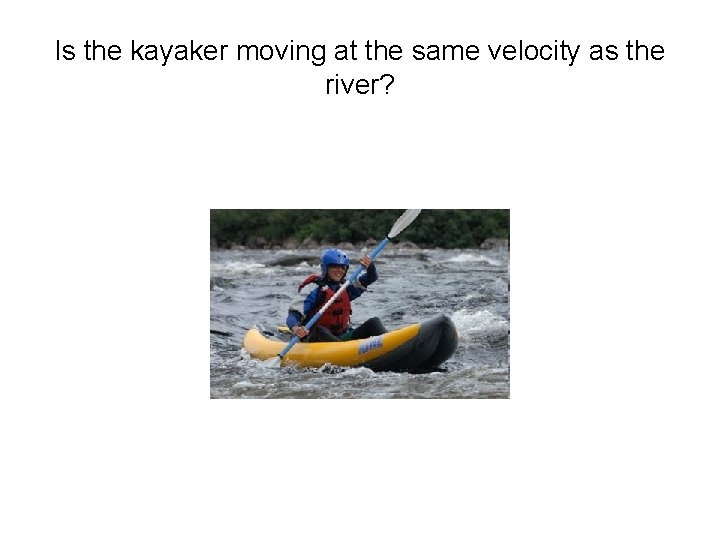Is the kayaker moving at the same velocity as the river?Chapter 5 Quiz 1 1. Give an example of a reference point. 2. What two things must you know to determine speed? a. time and direction b. direction and distance c. time and distance d. motion and direction 3. What is the difference between speed and velocity? a. speed uses direction and velocity does not b. velocity uses direction and speed does not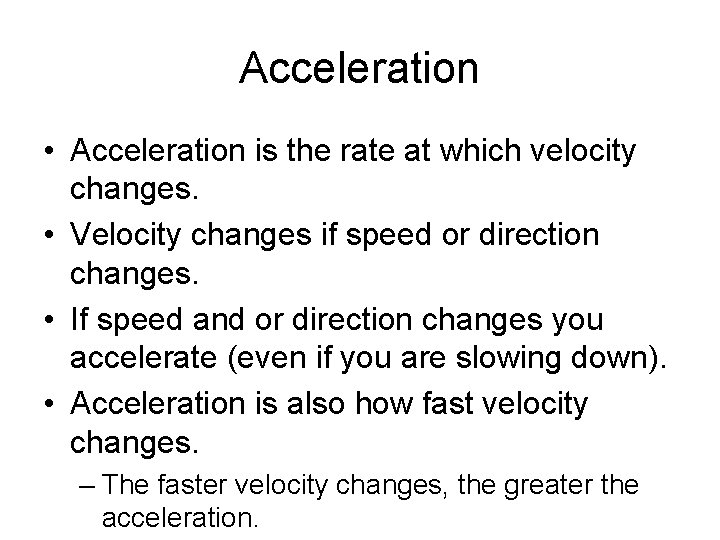Acceleration • Acceleration is the rate at which velocity changes. • Velocity changes if speed or direction changes. • If speed and or direction changes you accelerate (even if you are slowing down). • Acceleration is also how fast velocity changes. – The faster velocity changes, the greater the acceleration.Acceleration • Acceleration = final velocity – starting velocity time it takes to change velocity or Acceleration = change in velocity time Calculate Acceleration: A plane passes over Point A with a velocity of 8, 000 m/s north. Forty seconds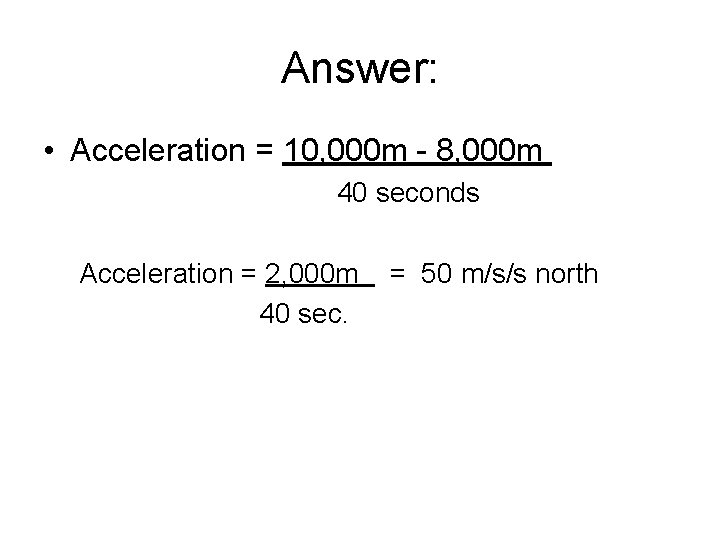Answer: • Acceleration = 10, 000 m - 8, 000 m 40 seconds Acceleration = 2, 000 m 40 sec. = 50 m/s/s north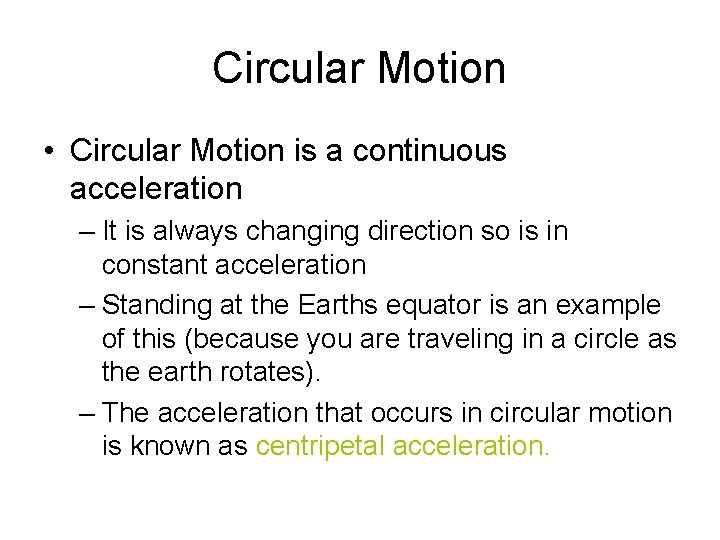Circular Motion • Circular Motion is a continuous acceleration – It is always changing direction so is in constant acceleration – Standing at the Earths equator is an example of this (because you are traveling in a circle as the earth rotates). – The acceleration that occurs in circular motion is known as centripetal acceleration.Chapter 5 Quiz 2 1. What is acceleration? a. the speed of an object b. the rate at which an object moves c. the rate at which time changes d. the rate at which velocity changes 2. Does a change in direction affect acceleration? 3. The acceleration that occurs in circular motion is known as: a. velocity acceleration b. motion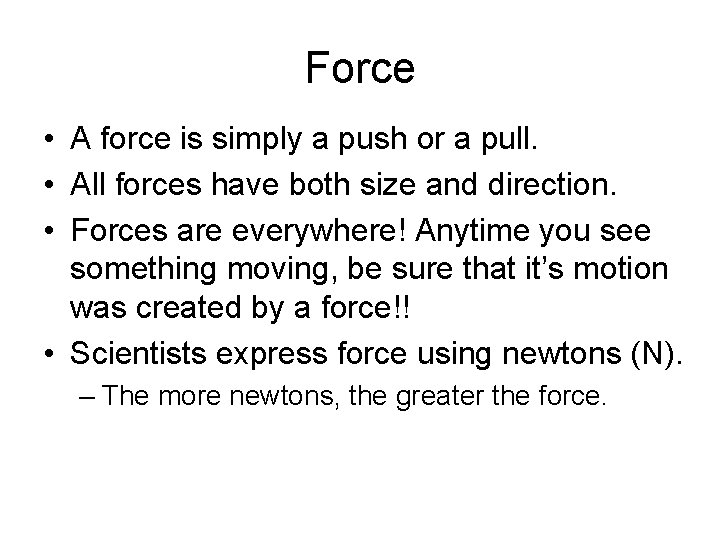Force • A force is simply a push or a pull. • All forces have both size and direction. • Forces are everywhere! Anytime you see something moving, be sure that it’s motion was created by a force!! • Scientists express force using newtons (N). – The more newtons, the greater the force.Force • The bulldozer is exerting a force on the pile of soil, but did you know that the pile of soil is also exerting a force, even though it is just sitting on the ground. • What objects in our classroom are exerting force, and which are receiving it?Forces in Combination • Often more than one force is exerted on an object at the same time. • The net force is the force that results from combining all the forces exerted on an object. • Example: when you are moving a piano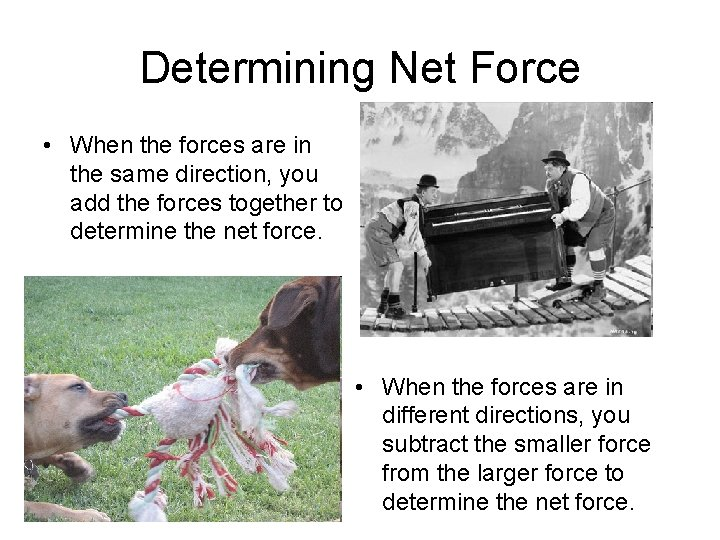Determining Net Force • When the forces are in the same direction, you add the forces together to determine the net force. • When the forces are in different directions, you subtract the smaller force from the larger force to determine the net force.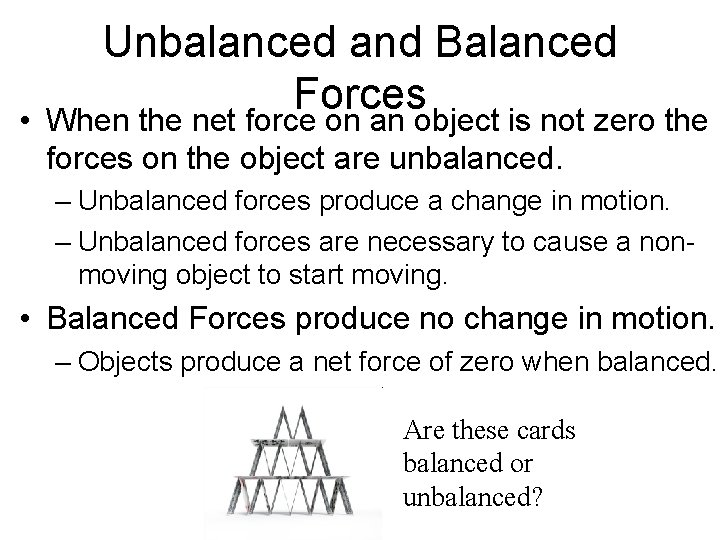• Unbalanced and Balanced Forces When the net force on an object is not zero the forces on the object are unbalanced. – Unbalanced forces produce a change in motion. – Unbalanced forces are necessary to cause a nonmoving object to start moving. • Balanced Forces produce no change in motion. – Objects produce a net force of zero when balanced. Are these cards balanced or unbalanced?Friction • Friction is a force that opposes motion between two surfaces that are touching • Friction occurs because the surface of any object is rough – Even surfaces that look smooth are covered with microscopic hills and valleys (see example)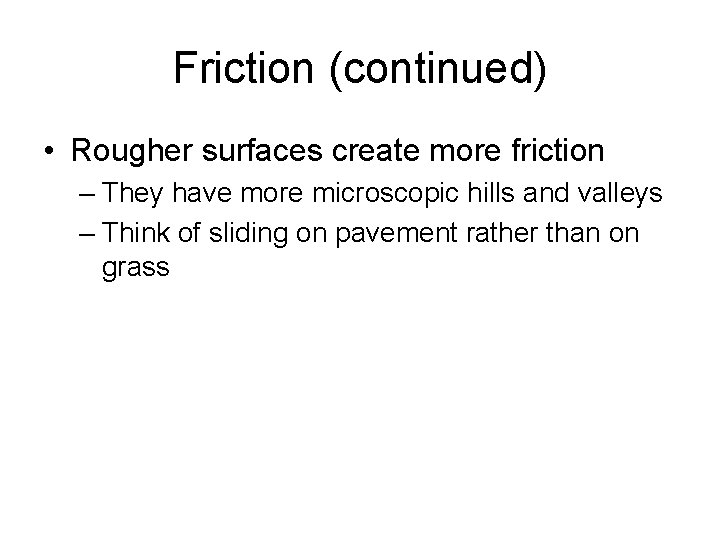Friction (continued) • Rougher surfaces create more friction – They have more microscopic hills and valleys – Think of sliding on pavement rather than on grass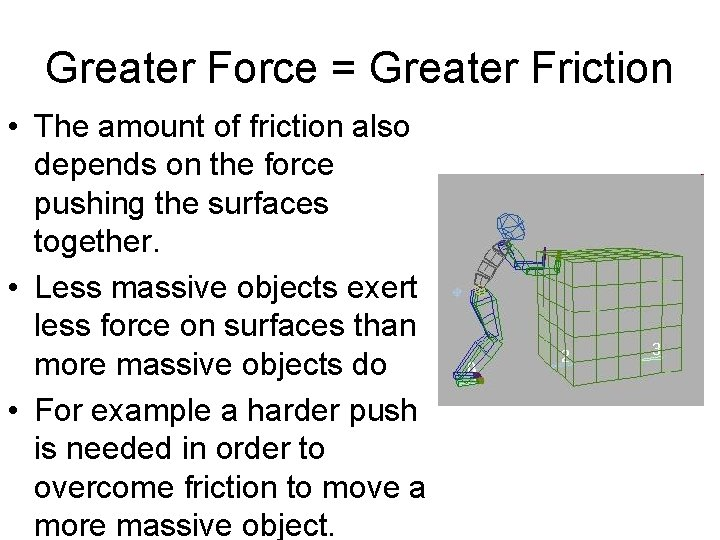Greater Force = Greater Friction • The amount of friction also depends on the force pushing the surfaces together. • Less massive objects exert less force on surfaces than more massive objects do • For example a harder push is needed in order to overcome friction to move a more massive object.Types of Friction • Sliding Friction: You use sliding friction when you go sledding, apply brakes on a bicycle, or when you write with a piece of chalk. The force of sliding friction is large. • Rolling Friction: usually requires less force than sliding friction, so is easier to move. Examples are anything with wheels. • Example: Sliding Friction-pushing furniture across floor. Rolling Friction-put wheels on furniture toTypes of Friction (cont) • Fluid Friction: Have you ever wondered why it is more difficult to walk on a freshly mopped floor vs. a dry one? This is because of Fluid Friction. • This is why swimming is such a good work out. You must exert force to overcome fluid friction.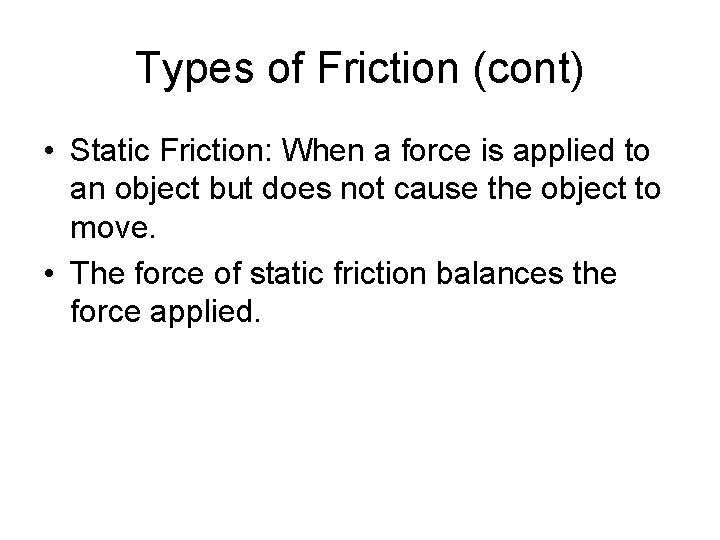Types of Friction (cont) • Static Friction: When a force is applied to an object but does not cause the object to move. • The force of static friction balances the force applied.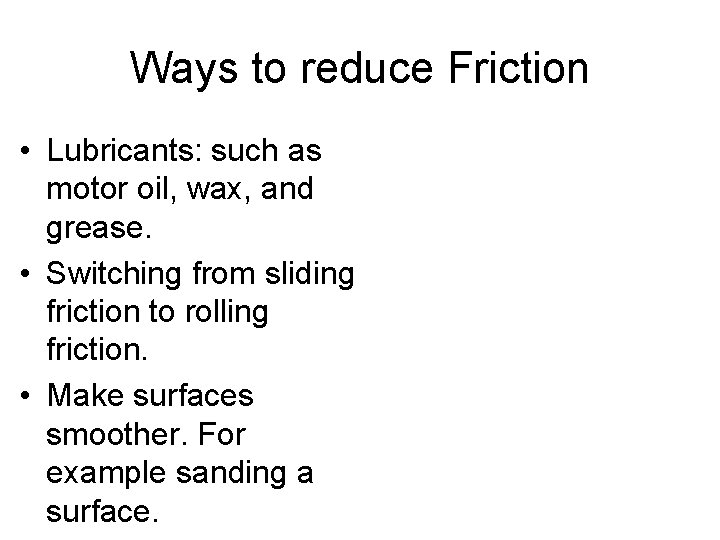Ways to reduce Friction • Lubricants: such as motor oil, wax, and grease. • Switching from sliding friction to rolling friction. • Make surfaces smoother. For example sanding a surface.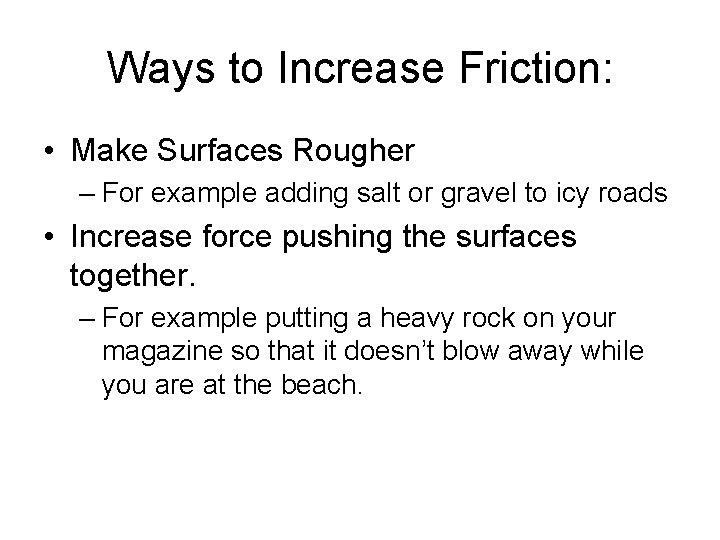Ways to Increase Friction: • Make Surfaces Rougher – For example adding salt or gravel to icy roads • Increase force pushing the surfaces together. – For example putting a heavy rock on your magazine so that it doesn’t blow away while you are at the beach.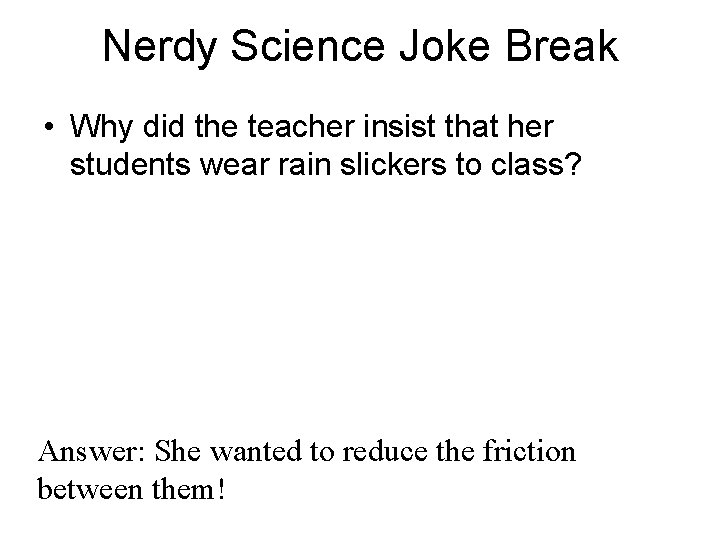Nerdy Science Joke Break • Why did the teacher insist that her students wear rain slickers to class? Answer: She wanted to reduce the friction between them!Friction Poetry • • • An impatient young girl named Lenore Tried to run on a freshly waxed floor. Since the friction was less, She made quite a mess As she slid right under the door.Chapter 5 Quiz 3 1. Friction is a force that: a. opposes an object’s motion b. does not exist when surfaces are very smooth c. decreases with larger mass. d. All of the above 2. Rolling friction a. is usually less than sliding friction b. makes it difficult to move objects on wheels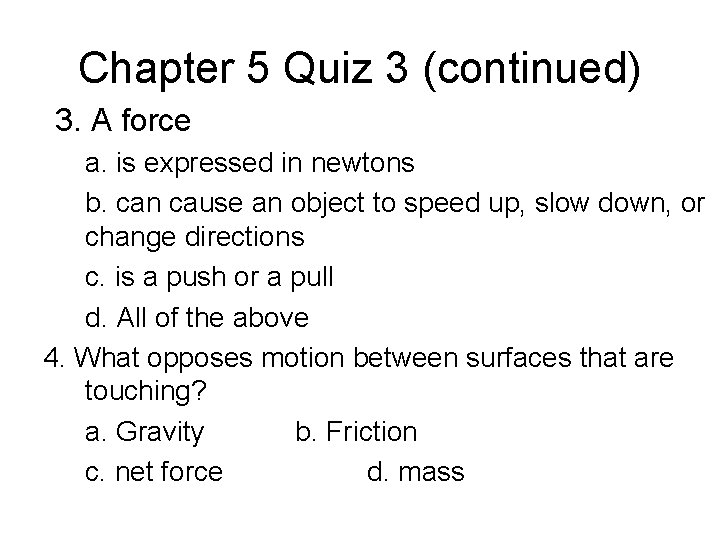Chapter 5 Quiz 3 (continued) 3. A force a. is expressed in newtons b. can cause an object to speed up, slow down, or change directions c. is a push or a pull d. All of the above 4. What opposes motion between surfaces that are touching? a. Gravity b. Friction c. net force d. massGravity • Gravity is a force of attraction between objects that is due to their masses. • All matter is affected by gravity – All matter has mass, and gravity is a result of mass. – All objects experience an attraction toward all other objects. – The greater the mass the stronger the attraction.Gravity on Earth • Earth’s gravitational force is large. – Compared to all other objects around you, Earth has the largest mass. – Earth’s gravitational force pulls everything toward the center of the Earth. • That is why objects in this room stay in place, and why dropped objects fall to the Earth rather than moving together or towards you.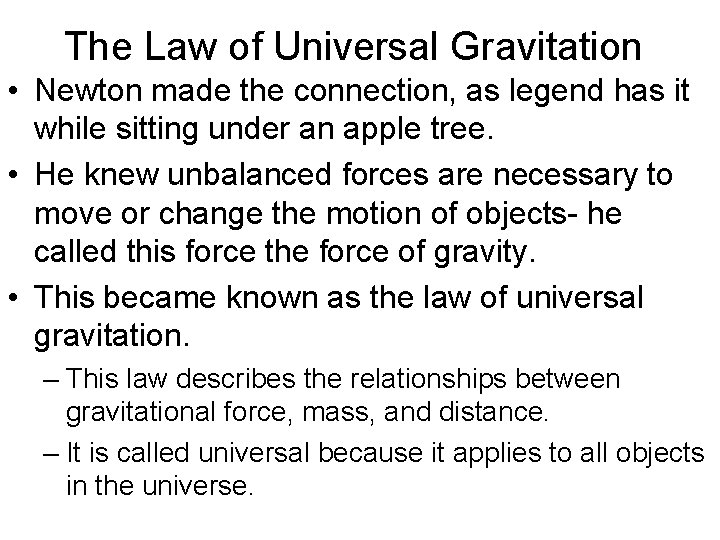The Law of Universal Gravitation • Newton made the connection, as legend has it while sitting under an apple tree. • He knew unbalanced forces are necessary to move or change the motion of objects- he called this force the force of gravity. • This became known as the law of universal gravitation. – This law describes the relationships between gravitational force, mass, and distance. – It is called universal because it applies to all objects in the universe.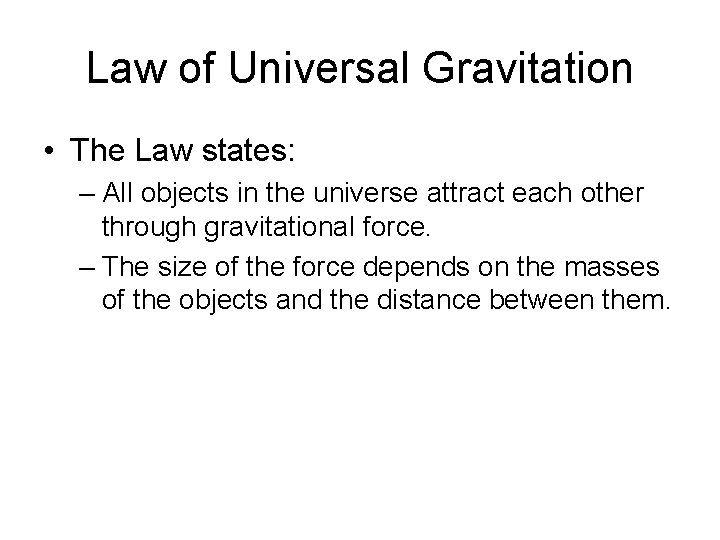Law of Universal Gravitation • The Law states: – All objects in the universe attract each other through gravitational force. – The size of the force depends on the masses of the objects and the distance between them.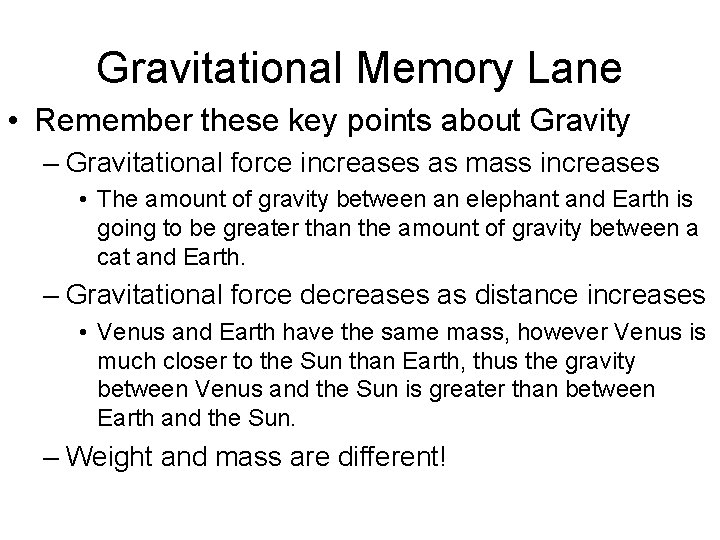Gravitational Memory Lane • Remember these key points about Gravity – Gravitational force increases as mass increases • The amount of gravity between an elephant and Earth is going to be greater than the amount of gravity between a cat and Earth. – Gravitational force decreases as distance increases • Venus and Earth have the same mass, however Venus is much closer to the Sun than Earth, thus the gravity between Venus and the Sun is greater than between Earth and the Sun. – Weight and mass are different!Chapter 5 Quiz 4 1. If Earth’s mass doubled, your weight would: a. increase because gravity increases b. decrease because gravity increases c. increase because gravity decreases d. not change because you are still on Earth 2. The amount of gravity between 1 kg of lead and Earth is _______ the amount of gravity between 1 kg of marshmallows and Earth a. greater than b. less than c. the same as d. None of the above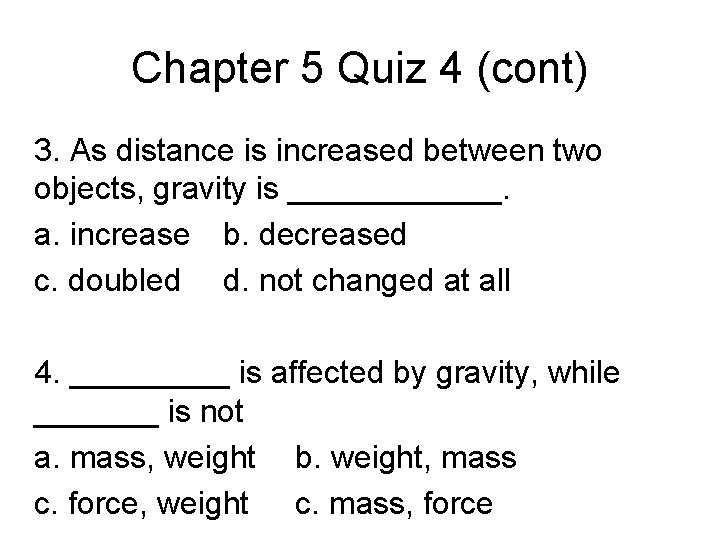Chapter 5 Quiz 4 (cont) 3. As distance is increased between two objects, gravity is ______. a. increase b. decreased c. doubled d. not changed at all 4. _____ is affected by gravity, while _______ is not a. mass, weight b. weight, mass c. force, weight c. mass, force